# Win in raffle

The raffle tickets were sold 200, 5 of which were winning. What is the probability that Peter, who bought one ticket will win?

Correct result:

p =  12.0051 %

#### Solution: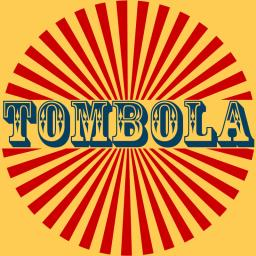We would be pleased if you find an error in the word problem, spelling mistakes, or inaccuracies and send it to us. Thank you!Tips to related online calculators
Would you like to compute count of combinations?

## Next similar math problems:

• Probability - tickets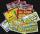What is the probability when you have 25 tickets in 5000 that you not wins the first (one) prize?
• Monty Hall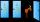Suppose you're on a game show, and you're given the choice of three doors: Behind one door is a car; behind the others, goats. You pick a door, say No. 1, and the host, who knows what's behind the doors, opens another door, say No. 3, which has a goat. Wh
• The testThe test contains four questions, and there are five different answers to each of them, of which only one is correct, the others are incorrect. What is the probability that a student who does not know the answer to any question will guess the right answer
• Raffle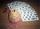How many raffle tickets must be purchased by Peter in raffle with issued 200 tickets if he wants to be sure win at least 3 price? In the raffle draws 30 prices.
• ThrowWe throw 2 times with 2 dices. What is the probability that the first roll will fall more than sum of 9 and the second throw have sum 3 or does not have the sum 4?
• DicesWe will throw two dice. What is the probability that the ratio between numbers on first and second dice will be 1:2?
• Sum on diceWe have two dice. What is the greater likelihood of fall a total sum 7 or 8 ? (write 7, 8 or 0 if the probabilities are the same)?
• Alarm systems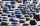What is the probability that at least one alarm system will signal the theft of a motor vehicle when the efficiency of the first system is 90% and of the independent second system 80%?
• Air draftThe numbers 1,2,3,4,5 are written on five tickets on the table. Air draft randomly shuffled the tickets and composed a 5-digit number from them. What is the probability that he passed: and, the largest possible number b, the smallest possible number c, a
• Sum 10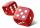What is the probability that two dice throw twice in a row will result the sum of 10?
• Peaches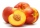There are 20 peaches in the pocket. 3 peaches are rotten. What is the probability that one of the randomly picked two peaches will be just one rotten?
• Probability of intersectionThree students have a probability of 0.7,0.5 and 0.4 to graduated from university respectively. What is the probability that at least one of them will be graduated?
• FamilyWhat is the probability that a family with 7 childrens have: exactly 5 girls? 7 girls and 0 boys? Consider the birth probability of a girl is 48.69% and boy 51.31%.
• Event probability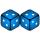The probability of event P in 8 independent experiments is 0.33. What is the probability that the event P occurs in one experiment (probability is same)?
• Three reds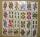What is the probability that when choosing 3 carats from seven carats, all 3 reds will be red?
• Sum or productWhat is the probability that two dice fall will have the sum 7 or product 12?
• PIN codePIN on Michael credit card is a four-digit number. Michael told this to his friend: • It is a prime number - that is, a number greater than 1, which is only divisible by number one and by itself. • The first digit is larger than the second. • The second d